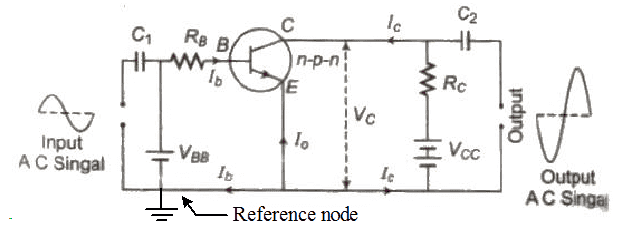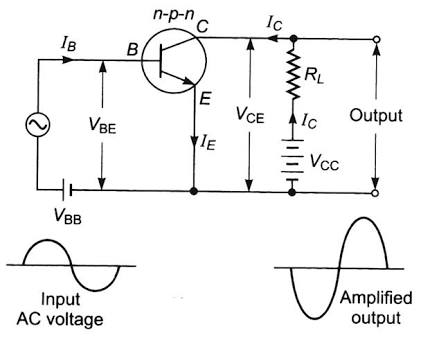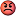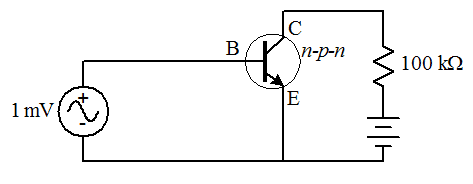# Transistor : Output voltage of the amplifier

Mentor
Suppose the lower terminal of battery VBB is earthed . Are you saying that the potential at left end of RB (where the current is entering ) is at a constant voltage VBB irrespective of the input AC signal ?
Yes, assuming that by "earthed" you mean that the bottom rail is taken to be the reference node.#### Attachments

•Jahnavi
Homework Helper
Gold Member
Ok sorry for me intervening out of nowhere BUT I think ##V_{BB}## will have some internal resistance so I think the transistor will see the input voltage after all...

Jahnavi
@gneill ,This picture is from another reference book . Here the author has put AC input signal in series with the DC battery . Does this look reasonable ?#### Attachments

•Delta2
Mentor
@gneill ,This picture is from another reference book . Here the author has put AC input signal in series with the DC battery . Does this look reasonable ?
Yes, that's a better representation. Note though that it doesn't depict an input resistor for the base in this case. That might not be a problem with careful choice of ##V_{BB}##.

•Jahnavi
Mentor
Ok sorry for me intervening out of nowhere BUT I think ##V_{BB}## will have some internal resistance so I think the transistor will see the input voltage after all...
Unless the problem states that the components are not ideal, it's not advisable to make that assumption. Further, you'd have no idea what size of resistance to ascribe to that internal resistance, so analyzing the circuit would be problematical.

Jahnavi
Yes, that's a better representation. Note though that it doesn't depict an input resistor for the base in this case. That might not be a problem with careful choice of ##V_{BB}##.

Ah ! Finally.

But the irony is that in the exams I need to stick with the diagram in post #31 however flawed it might be. ( It is the standard reference text)

Thank you for your patience .Please bear with me for some more time . I have few more relevant enquiries .

Jahnavi
Yes, that's a better representation. Note though that it doesn't depict an input resistor for the base in this case. That might not be a problem with careful choice of ##V_{BB}##.

In the picture in post#38 , the DC voltage from battery and applied AC input signal get added ?

Is the net input voltage in the Base sinusoidal but the peak voltage now oscillating between VBB+Vo to VBB-Vo ?

Homework Helper
Gold Member
Ok @Jahnavi maybe in the text of the book mentions something like that all DC voltage sources are assumed to have a (small) internal resistance?

•cnh1995
Mentor
In the picture in post#38 , the DC voltage from battery and applied AC input signal get added ?
Yes.
Is the net input voltage in the Base sinusoidal but the peak voltage now oscillating between VBB+Vo to VBB-Vo ?
Yes, it's an AC signal with a DC offset. Mathematically you could write it as:

##V_{BE}(t) = V_{BB} + V_o sin(\omega t)##

•Jahnavi
Jahnavi
Yes, it's an AC signal with a DC offset. Mathematically you could write it as:

##V_{BE}(t) = V_{BB} + V_o sin(\omega t)##

Thanks .

What happens to the net input voltage in the original problem (post #1 where there is no coupling capacitor ) ?

I am referring to the case when AC input signal is applied across the branch having resistor and DC battery .

Homework Helper
Gold Member
Thanks .

What happens to the net input voltage in the original problem (post #1 where there is no coupling capacitor ) ?

I am referring to the case when AC input signal is applied across the branch having resistor and DC battery .
Base-emitter voltage will be equal to the input ac voltage and the base current will have a half wave rectified waveform.
Plus, the battery will add a dc component in the ac source current.
Edit: I was assuming BE junction to be ideal (0V drop).If the BE junction is assumed to have 0.7V drop and if the ac source is of 1mV, then, as gneill said, it won't be able to turn the transistor on.

Last edited:
•Delta2
Mentor
Thanks .

What happens to the net input voltage in the original problem (post #1 where there is no coupling capacitor ) ?

I am referring to the case when AC input signal is applied across the branch having resistor and DC battery .
The base DC bias circuit (battery and resistor branch) is rendered ineffective by the placement of the input source, so an equivalent circuit as far as the transistor is concerned would be:Now, ##1\;mV## is not enough to forward bias the transistor, which needs about ##700\;mV## for a silicon transistor. Without a proper base bias to place the transistor operating point in the appropriate location on its ##IV## characteristic curves, the transistor would never turn on and no signal would pass.

#### Attachments

•Jahnavi and cnh1995
Jahnavi
The base DC bias circuit (battery and resistor branch) is rendered ineffective by the placement of the input source,

Does that mean there would be no current in the branch consisting of the 2kΩ resistor and DC battery ?

Why does AC input voltage dominate over the DC battery so as to make it ineffective ?

Mentor
Does that mean there would be no current in the branch consisting of the 2kΩ resistor and DC battery ?
No, the AC source will provide a path for it. KVL around the loop will show that the majority of the bias will be dropped across the resistor.
Why does AC input voltage dominate over the DC battery so as to make it ineffective ?
Any ideal source, AC or DC, would do the same. The point is, what ever source you place in that position will set the potential difference between the two ends of that "bias" branch.

•Jahnavi
Jahnavi
Thanks !